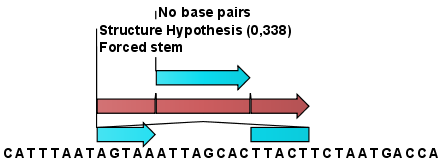## Probabilities

After evaluation of the structure hypothesis an annotation is added to the input sequence. This annotation covers the same region as the annotations that constituted the hypothesis and contains information about the probability of the evaluated hypothesis (see figure 23.24).Figure 23.24: This hypothesis has a probability of 0.338 as shown in the annotation.